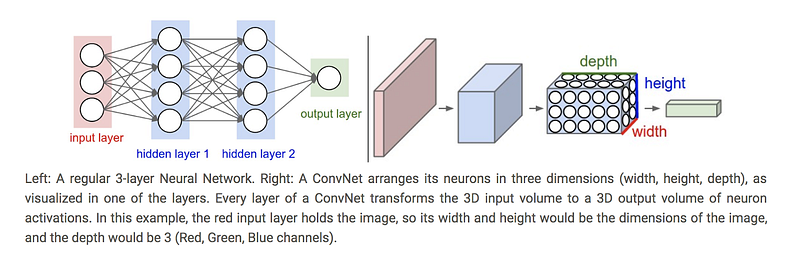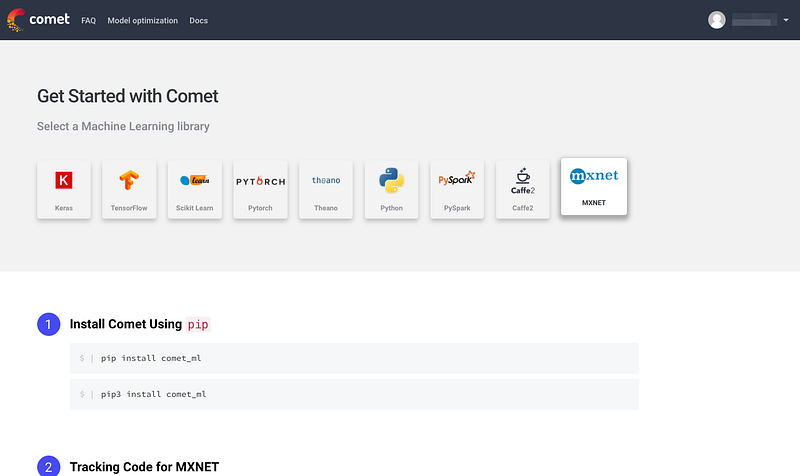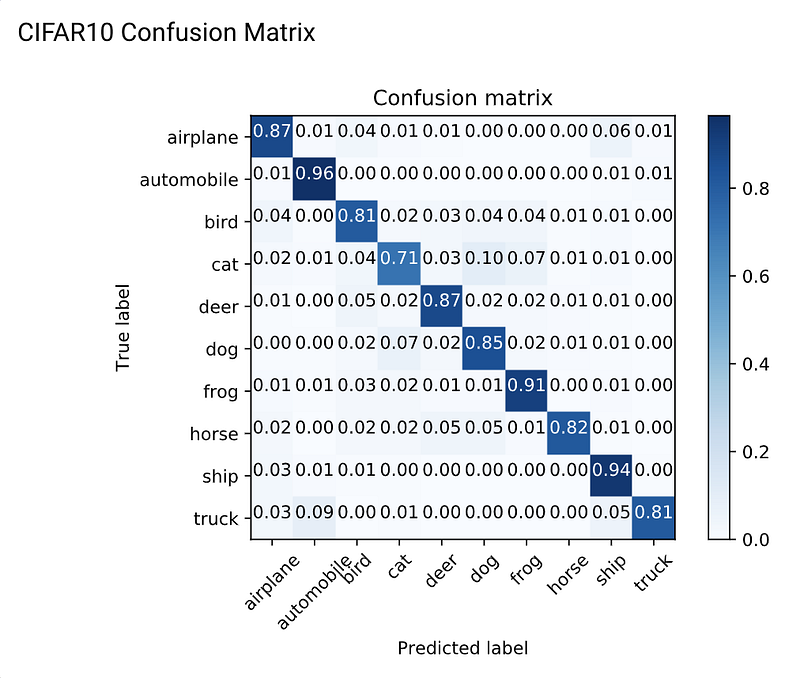# Implementing ResNet with MXNET Gluon and Comet.ml for image classificationIn this tutorial, we will illustrate how to build an image recognition model using a convolutional neural network (CNN) implemented in MXNet Gluon, and integrate Comet.ml for experiment tracking and monitoring. We will be using the MXNet ResNet model architecture and training that model on the CIFAR 10 dataset for our image classification use case.

MXNet is a deep learning framework that offers optimizations for multi-GPU training and accelerates model development with predefined layers and automatic differentiation. Since MXNet was constructed with large-scale neural networks in mind, the framework is designed for both efficiency and flexibility.

Whether MXNet is an entirely new framework for you or you have used the MXNet backend while training your Keras models, this tutorial illustrates how to build an image recognition model with an MXNet resnet_v1 model.

#### About the model architecture

CNNs are a specific category of neural networks that work well with data that has a spatial relationship, making them popular for text data and time-series data, in addition to image data. CNNs are basically just several layers of convolutions with nonlinear activation functions like ReLU or tanh applied to the results. Unlike other neural networks that use fully connected layers, CNNs use convolutions over the input layer to compute the output. This results in local connections, where each region of the input is connected to a neuron in the output. This architecture greatly reduces the number of parameters in the network and allows for a more efficient forward function implementation.Stanford’s CS231 course has an excellent overview of CNNs here as does Denny Britz’s blog

We are using the ResNet CNN architecture for our tutorial because it allows us to have a deeper architecture without sacrificing performance due to the vanishing gradient problem. It solves this problem by introducing “shortcut connections” to fit the input from the previous layer to the next layer without any modification to the input. ResNet’s claim to fame was winning 1st place in the ILSVRC 2015 classification competition and appeared in the He et. al 2015 paper. Although ResNet was originally used for the ImageNet problem, for this tutorial, we’re adapting it to CIFAR-10.

#### About the data

The CIFAR 10 dataset is a labeled subset of the 80 million tiny images dataset It contains 10 mutually exclusive classes (including dogs, cats, planes, automobiles, planes, etc…) with 6,000 images per class. The dataset is divided into five training batches and one test batch so that the test batch contains 1,000 randomly-selected images from each class.

During our training experiments, we’ll explore and adjust different hyperparameters. Since each training session for a particular hyperparameter configuration creates a new model, it becomes harder to manage these as we make progress. This tutorial will also show the use of Comet.ml which allows us to monitor and track our experimentation work.

#### Tutorial Step Overview

We will provide a step-by-step overview next, but if you would like to download the entire script, you can do so here.

1. First we’ll need to make sure we have the right environment to run the training code:

Hardware: We recommend using a machine with at least 1 GPU to expedite the training process. If you don’t have a GPU available you can also train it on a CPU but training will be very slow. Simply set the num_gpu argument to 0 when you run the script and it will default to using CPUs. See an example command below:

`python mxnet_cifar10.py --num-epochs 240 --mode hybrid --num-gpus 0 -j 8 --batch-size 128 --wd 0.0001 --lr 0.1 --lr-decay 0.1 --lr-decay-epoch 80,160 --model cifar_resnet20_v1`
• Software: Make sure your environment has the proper library versions installed:
• Install MXNet by following the instructions available here
• Install GluonCV by running
`pip install gluoncv --upgrade`
• Install Comet-ml by running
`pip install comet_ml`

Next, we’ll set up a Comet account and project so we can track the results of our different model iterations. Go to www.comet.ml and sign up with either your email address or Github account.

Once you select a plan, you will see a project Quickstart Guide that contains your Comet API key for your projectEasy install instructions for Comet in the Quick Start Guide

Now that we have our environment ready we can finally start building our model! Note that the steps we just went through will only need to happen once.

#### Code overview:

We’ll begin by importing with the different libraries we need. Make sure to import comet_ml at the top of your file. We also define the Comet experiment at the top with the API Key you obtained in Step 2 above and your project and workshop name.

`from comet_ml import Experimentimport argparseimport timeimport loggingimport numpy as npimport mxnet as mxfrom mxnet import gluon, ndfrom mxnet import autograd as agfrom mxnet.gluon import nnfrom mxnet.gluon.data.vision import transformsfrom gluoncv.model_zoo import get_modelfrom gluoncv.utils import makedirs, TrainingHistoryfrom gluoncv.data import transforms as gcv_transformsfrom sklearn.metrics import confusion_matriximport itertoolsimport matplotlib.pyplot as pltplt.switch_backend('agg')experiment = Experiment(api_key="<YOUR API KEY>", project_name="mxnet-comet-tutorial", workspace="<YOUR WORKSPACE>")`

As noted in Step 1, we recommend using at least 1 GPU to train this model. Set the – -num_gpus = 1 and define the context to establish what GPU to use.

`batch_size = opt.batch_sizeclasses = 10class_labels = ['airplane', 'automobile', 'bird', 'cat','deer', 'dog', 'frog', 'horse', 'ship', 'truck']`
`num_gpus = opt.num_gpusbatch_size *= max(1, num_gpus)context = [mx.gpu(i) for i in range(num_gpus)] if num_gpus > 0 else [mx.cpu()]num_workers = opt.num_workers`

Next, we’ll set up our data augmentation. Data augmentation both increases the amount of training data and is a great technique for reducing overfitting on models. For more details on data augmentation in gluon, you can refer to this tutorial. Here, we’ll be resizing, cropping, flipping, adjusting the lighting, and using other augmentation techniques on our CIFAR-10 dataset. These transformation operations will be randomized for training, but not during our prediction step.

`transform_train = transforms.Compose([gcv_transforms.RandomCrop(32,pad=4),transforms.RandomFlipLeftRight(),transforms.ToTensor(),transforms.Normalize([0.4914, 0.4822, 0.4465],[0.2023, 0.1994, 0.2010])])transform_test = transforms.Compose([transforms.ToTensor(),transforms.Normalize([0.4914, 0.4822, 0.4465], [0.2023, 0.1994, 0.2010])])`

For our learning rate, we define a decay factor, lr_decay, and the epochs where the learning rate decays. We pay special attention to the learning rate since it controls how much we are adjusting the weights of our network with respect to the loss gradient and ultimately can have an impact on how quickly our model converges to a local minima. A good learning rate can help cut down on the time it takes to train the model.

`lr_decay = opt.lr_decaylr_decay_epoch = [int(i) for i in opt.lr_decay_epoch.split(',')] + [np.inf]`

For our model, we’re using the cifar_restnet20_v1 model architecture available from the MXNet gluoncv model zoo. We’ll use the Nesterov accelerated gradient descent algorithm for our optimizer. Nesterov accelerated gradient uses a “gamble, correct” approach to updating gradients where it uses momentum and the previously calculated gradient to make an informed update to the next gradient that can be corrected later. You can read more about the Nesterov accelerated gradient here.

We can also set the cadence at which our model saves with save_period.

`model_name = opt.modelif model_name.startswith('cifar_wideresnet'):     kwargs = {'classes': classes,'drop_rate': opt.drop_rate}else:     kwargs = {'classes': classes}net = get_model(model_name, **kwargs)`
`if opt.resume_from:      net.load_parameters(opt.resume_from, ctx = context)optimizer = 'nag'save_period = opt.save_periodif opt.save_dir and save_period:      save_dir = opt.save_dir      makedirs(save_dir)else:       save_dir = ''       save_period = 0plot_path = opt.save_plot_dirlogging.basicConfig(level=logging.INFO)logging.info(opt)`

Now we’ll actually split out the data and labels for the validation dataset and define our model evaluation metrics around accuracy.

`def test(ctx, val_data):     metric = mx.metric.Accuracy()     for i, batch in enumerate(val_data):           data = gluon.utils.split_and_load(batch, ctx_list=ctx, batch_axis=0)           label = gluon.utils.split_and_load(              batch, ctx_list=ctx, batch_axis=0)           outputs = [net(X) for X in data]      metric.update(label, outputs)return metric.get()`

Finally, we’ll implement the function for training our model. Our train function includes the data loaders for our train and validation data. Since we’re dealing with a single label, multi-class classification problem, we will use the softmax cross entropy loss function.

You’ll notice we added in a line around tracking our model’s training error and validation error.

`experiment.log_multiple_metrics({"acc":acc,"val_acc":val_acc})`

See the full training function here:

`def train(epochs, ctx):if isinstance(ctx, mx.Context):            ctx = [ctx]      net.initialize(mx.init.Xavier(), ctx=ctx)train_data = gluon.data.DataLoader(gluon.data.vision.CIFAR10(train=True).transform_first(transform_train), #set path to the downloaded databatch_size=batch_size, shuffle=True, last_batch='discard', num_workers=num_workers)val_data = gluon.data.DataLoader(gluon.data.vision.CIFAR10(train=False).transform_first(transform_test), batch_size=batch_size, shuffle=False, num_workers=num_workers) trainer = gluon.Trainer(net.collect_params(), optimizer {'learning_rate': opt.lr, 'wd': opt.wd, 'momentum': opt.momentum})        metric = mx.metric.Accuracy()        train_metric = mx.metric.Accuracy()        loss_fn = gluon.loss.SoftmaxCrossEntropyLoss()iteration = 0lr_decay_count = 0best_val_score = 0`
`for epoch in range(epochs):         tic = time.time()         train_metric.reset()         metric.reset()         train_loss = 0         num_batch = len(train_data)         alpha = 1       if epoch == lr_decay_epoch[lr_decay_count]:               new_lr =trainer.learning_rate*lr_decay               trainer.set_learning_rate(new_lr)               experiment.log_metric("lr",new_lr)               lr_decay_count += 1for i, batch in enumerate(train_data):           data = gluon.utils.split_and_load(batch, ctx_list=ctx, batch_axis=0)           label = gluon.utils.split_and_load(batch, ctx_list=ctx, batch_axis=0)         with ag.record():                 output = [net(X) for X in data]                 loss = [loss_fn(yhat, y) for yhat, y in zip(output, label)]for l in loss:                 l.backward()                 trainer.step(batch_size)                 train_loss += sum([l.sum().asscalar() for l in loss])                 train_metric.update(label, output)                 name, acc = train_metric.get()                 iteration += 1          train_loss /= batch_size * num_batch          name, acc = train_metric.get()          name, val_acc = test(ctx, val_data)      experiment.log_multiple_metrics({"acc":acc,"val_acc":val_acc})    if val_acc > best_val_score:           best_val_score = val_accnet.save_parameters('%s/%.4f-cifar-%s-%d-best.params'%(save_dir, best_val_score, model_name, epoch))            name, val_acc = test(ctx, val_data)            logging.info('[Epoch %d] train=%f val=%f loss=%f time: %f' % (epoch, acc, val_acc, train_loss, time.time()-tic))     if save_period and save_dir and (epoch + 1) % save_period == 0:            net.save_parameters('%s/cifar10-%s-%d.params'%(save_dir, model_name, epoch))     if save_period and save_dir:            net.save_parameters('%s/cifar10-%s-%d.params'%(save_dir, model_name, epochs-1))def main():if opt.mode == 'hybrid':         net.hybridize()    train(opt.num_epochs, context)﻿if __name__ == '__main__':     main()`

Now you can run your model script with first set of parameters and arguments. If you’d like to test before running it for the full 240 epochs, you can set the num_epochs argument to smaller number (for example, 3 epochs).

`python cifar_10_train.py --num-epochs 240 --mode hybrid --num-gpus 1 -j 8 --batch-size 64 --wd 0.0001 --lr 0.1 --lr-decay 0.1 --lr-decay-epoch 80,160 --model cifar_resnet20_v1`

You will see a message at the start of the output that will indicate where your Comet experiment is being logged (see a similar screenshot below). Click on this experiment url to see your model training results.

#### Monitoring results inside the Comet UI

As an example, we’ve logged the results in a public Comet project: https://www.comet.ml/ceceshao1/mxnet-comet-tutorial

• We can actually observe the training and validation accuracy plots update in real-time as results come in.
• We also want to make sure we’re actually using our GPU, so we can go to the System Metrics tab to check memory usage and utilization.
• The script we ran and the output we saw after running it can be found on the Code and Output tabs, respectively.
• You’ll see some noticeable bumps in accuracy at epoch 80 because we set our learning rate decay to occur at epoch 80 and 160. For our next model iteration, we can test to see what happens when we adjust our learning rate decay cadence.
• For classification problems, it’s very useful to plot a confusion matrix to see the correct and incorrect predictions for each class. The script you can download here (and at the beginning of the tutorial) includes the functions to create a confusion matrix. We also log the confusion matrix as a figure to our Comet.ml experiment once the model finishes running.
`experiment.log_figure(figure_name=’CIFAR10 Confusion Matrix’, figure=plt)`Some examples of a class where our model made a higher proportion of incorrect predictions was mistaking trucks with automobiles or dogs for horses. Simply look at the higher values in the confusion matrix to identify where the model can be improved (perhaps by collecting more data around these specific classes)

Our first model performed very well with a high training accuracy around 0.9941. However, when we take a look at the validation accuracy of 0.9148 it’s clear that our model is overfitting. We could introduce dropout to eliminate some of this overfitting, but it would come at a cost to accuracy

#### Another model iteration

Next, try running the script with a second set of parameters — this time, we will increase the batch size to 128 and our learning rate decay cadence to the 40th and 100th epoch to see how that impacts performance.

`python mxnet_cifar10.py — num-epochs 240 — mode hybrid — num-gpus 1 -j 8 — batch-size 128 — wd 0.0001 — lr 0.1 — lr-decay 0.1 — lr-decay-epoch 40,100 — model cifar_resnet20_v1`

#### Compare results in Comet.ml

This second run will be logged as a different Comet experiment. Having the two experiments in the same project will allow us to begin conducting meta-analysis on our model iterations with higher-level visualizations and queries.

Our second experiment has significantly worse results with a training accuracy of 0.852 and a validation accuracy of 0.8184. Back to the drawing board…

You can check the exact differences between the experiments by selecting the two experiments and pressing ‘Diff’ — see how the code diffs look between our experiments here and in the screenshot below.

#### Quick Recap

We hope this tutorial serves as a good starting point for building an image classification model using MXNet Gluon with Comet.ml. To summarize the tutorial highlights, we:

• Trained a MXNet Gluon resnet_v1 model on the CIFAR-10 dataset to learn how to classify images into 10 classes using this script
• Explored a different iteration of the model training experiment where we increased the batch size and compared our two versions in Comet.ml
• Used Comet.ml to automatically capture our model’s results (training accuracy and validation accuracy), training code, and other artifacts

You can see a quick start guide on Comet.ml here and learn more about MXNet here.

Bonus Notes: